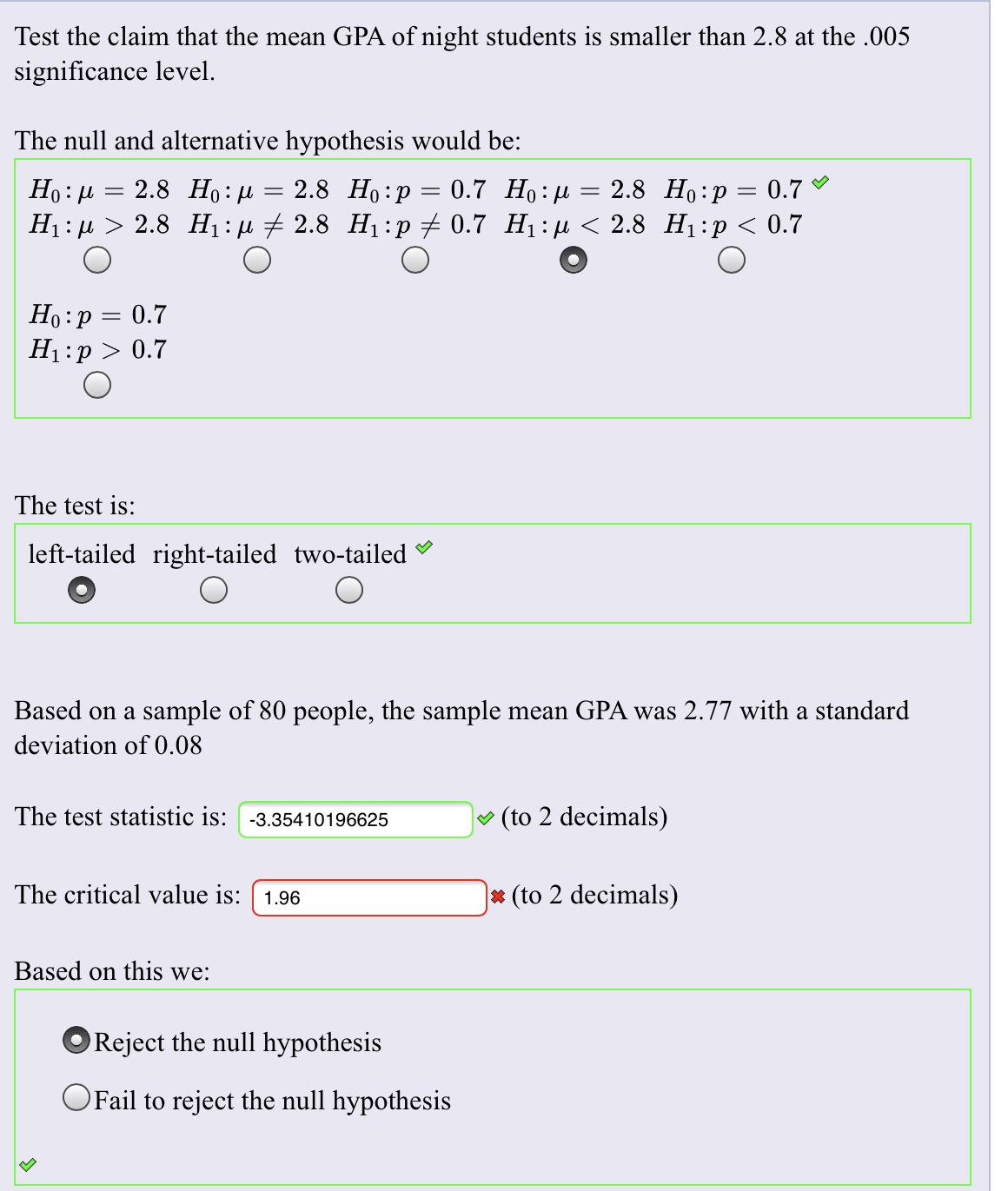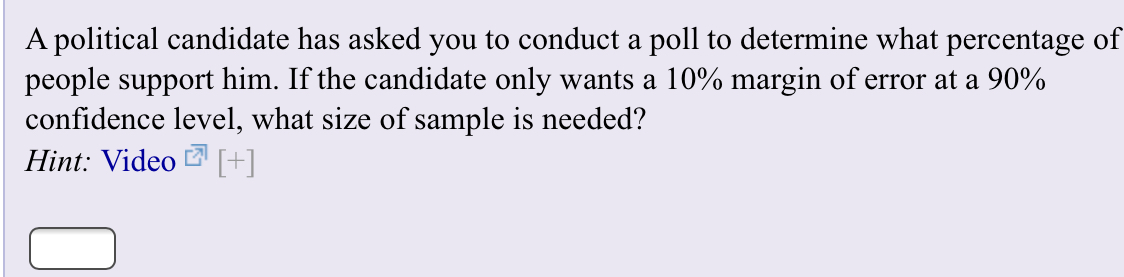# Test the claim that the mean GPA of night students is smaller than 2.8 at the .005significance level.The null and alternative hypothesis would be:0.7 Но: и — 2.8 Но: р — 0.72.8 H p 0.7Ho 2.8 Ho: u 2.8 Ho:pH u 2.8 H1 2.8 H1 p 0.7 H11Но:р 3 0.7H1 p0.7The test isleft-tailed right-tailed two-tailedBased on a sample of 80 people, the sample mean GPA was 2.77 with a standarddeviation of 0.08The test statistic is: -3.35410196625(to 2 decimals)The critical value is: 1.96* (to 2 decimals)Based on this we:Reject the null hypothesisOFail to reject the null hypothesis A political candidate has asked you to conduct a poll to determine what percentage ofpeople support him. If the candidate only wants a 10% margin of error at a 90%confidence level, what size of sample is needed?Hint: Video []

Question
104 views

I need help finding the answer on the TI84 Plus CE and on paper ?. Thank youhelp_outlineImage TranscriptioncloseTest the claim that the mean GPA of night students is smaller than 2.8 at the .005 significance level. The null and alternative hypothesis would be: 0.7 Но: и — 2.8 Но: р — 0.7 2.8 H p 0.7 Ho 2.8 Ho: u 2.8 Ho:p H u 2.8 H1 2.8 H1 p 0.7 H1 1 Но:р 3 0.7 H1 p0.7 The test is left-tailed right-tailed two-tailed Based on a sample of 80 people, the sample mean GPA was 2.77 with a standard deviation of 0.08 The test statistic is: -3.35410196625 (to 2 decimals) The critical value is: 1.96 * (to 2 decimals) Based on this we: Reject the null hypothesis OFail to reject the null hypothesis fullscreenhelp_outlineImage TranscriptioncloseA political candidate has asked you to conduct a poll to determine what percentage of people support him. If the candidate only wants a 10% margin of error at a 90% confidence level, what size of sample is needed? Hint: Video [] fullscreen
check_circle

Step 1

Alternate hypotheses Ha are generally the claims we are testing. Our claim is that the mean GPA<2.8.

H0: Mean = 2.8

Ha: Mean <2.8

Step 2

From the alternate hypotheses, we can see that the test is one-sided. The sign of hypothesis claim tells us if the test is left-sided or right-sided.

< sign hints at left-sided test

> sign means right-tailed test

Step 3

Test statistic can be  calculated ...

### Want to see the full answer?

See Solution

#### Want to see this answer and more?

Solutions are written by subject experts who are available 24/7. Questions are typically answered within 1 hour.*

See Solution
*Response times may vary by subject and question.
Tagged in

### Measures